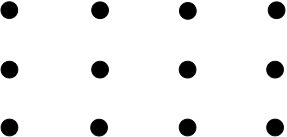# Counting Dots in Arrays

Alignments to Content Standards: 2.OA.C.4

Which of the following are equal to the number of dots in the picture below? (Choose all that apply.)1. 3 + 3 + 3
2. 3 + 4
3. 4 + 4 + 4
4. 4 + 4 + 4 + 4
5. 3 + 3 + 3 + 3

## IM Commentary

Students who work on this task will benefit in seeing that given a quantity, there is often more than one way to represent it, which is a precursor to understanding the concept of equivalent expressions. This particular question also lays a foundation for students to understand the commutative property of multiplication in third grade. This task would be much more valuable if included in an appropriate place in an instructional sequence than as an isolated task.

## Solution

We can see 3 rows with 4 dots in each row, so (c) 4+4+4 can represent the number of dots in the array. We can also see 4 columns with 3 dots in each column, so (e) 3+3+3+3 can represent the number of dots in the array.